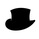17181 просмотров
17181
```study("ADX and DI")
len = input(title="Length", type=integer, defval=3)
len1 = input(title="Length1", type=integer, defval=1)
th = input(title="threshold", type=integer, defval=20)

TrueRange = max(max(high-low, abs(high-nz(close))), abs(low-nz(close)))
DirectionalMovementPlus = high-nz(high) > nz(low)-low ? max(high-nz(high), 0): 0
DirectionalMovementMinus = nz(low)-low > high-nz(high) ? max(nz(low)-low, 0): 0

SmoothedTrueRange = nz(SmoothedTrueRange) - (nz(SmoothedTrueRange)/len) + TrueRange
SmoothedDirectionalMovementPlus = nz(SmoothedDirectionalMovementPlus) - (nz(SmoothedDirectionalMovementPlus)/len) + DirectionalMovementPlus
SmoothedDirectionalMovementMinus = nz(SmoothedDirectionalMovementMinus) - (nz(SmoothedDirectionalMovementMinus)/len) + DirectionalMovementMinus

DIPlus = SmoothedDirectionalMovementPlus / SmoothedTrueRange * 100
DIMinus = SmoothedDirectionalMovementMinus / SmoothedTrueRange * 100
DX = abs(DIPlus-DIMinus) / (DIPlus+DIMinus)*100
//Skuska
crosscall = (DIPlus > DIMinus)
crossput = (DIMinus > DIPlus)
DIPcross = sma(crosscall, len1)
DIPput = sma(crossput, len1)
DIPOVB = (DIPlus > 60)
DIMOVS = (DIMinus > 60)

bgcolor(DIMOVS ? lime : na, transp=40)
bgcolor(DIPOVB ? orange : na, transp=20)
plot(DIPlus, color=green, linewidth=3, title="DI+")
plot(DIMinus, color=red, linewidth=3, title="DI-")